### 14. GENERALIZED MAXIMUM-LIKELIHOOD METHOD

So far we have always worked with the standard maximum-likelihood formalism, whereby the distribution functions are always normalized to unity. Fermi has pointed out that the normalization requirement is not necessary so long as the basic principle is observed: namely, that if one correctly writes down the probability of getting his experimental result, then this likelihood function gives the relative probabilities of the parameters in question. The only requirement is that the probability of getting a particular result be correctly written. We shall now consider the general case in which the probability of getting an event in dx is F(x)dx, and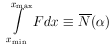is the average number of events one would get if the same experiment were repeated many times. According to Eq. (19), the probability of getting no events in a small finite intervalx is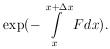The probability of getting no events in the entire interval xmin < x < xmax is the product of such exponentials or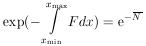The element of probability for a particular experimental result of N events at x = x1, ... , xN is then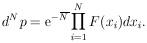Thus we have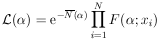and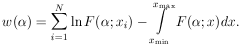The solutionsi =i* are still given by the M simultaneous equations:The errors are still given by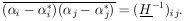whereThe only change is that N no longer appears explicitly in the formula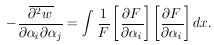A derivation similar to that used for Eq. (8) shows that N is already taken care of in the integration over F(x).

In a private communication, George Backus has proven, using direct probability, that the Maximum-Likelihood Theorem also holds for this generalized maximum-likelihood method and that in the limit of large N there is no method of estimation that is more accurate. Also see Sect. 9.8 of Ref. 6.

In the absence of the generalized maximum-likelihood method our procedure would have been to normalize F(; x) to unity by using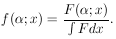For example, consider the sample containing just two radioactive species, of lifetimes1 and2. Let3 and4 be the two initial decay rates. Then we have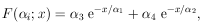where x is the time. The standard method would then be to use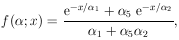which is normalized to one. Note that the four original parameters have been reduced to three by using54 /3. Then3 and4 would be found by using the auxiliary equation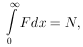the total number of counts. In this standard procedure the equation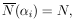must always hold. However, in the generalized maximum-likelihood method these two quantities are not necessarily equal. Thus the generalized maximum-likelihood method will give a different solution for thei, which should, in principle, be better.

Another example is that the best value for a cross sectionis not obtained by the usual procedure of settingL = N (the number of events in a path length L). The fact that one has additional prior information such as the shape of the angular distribution enables one to do a somewhat better job of calculating the cross section.